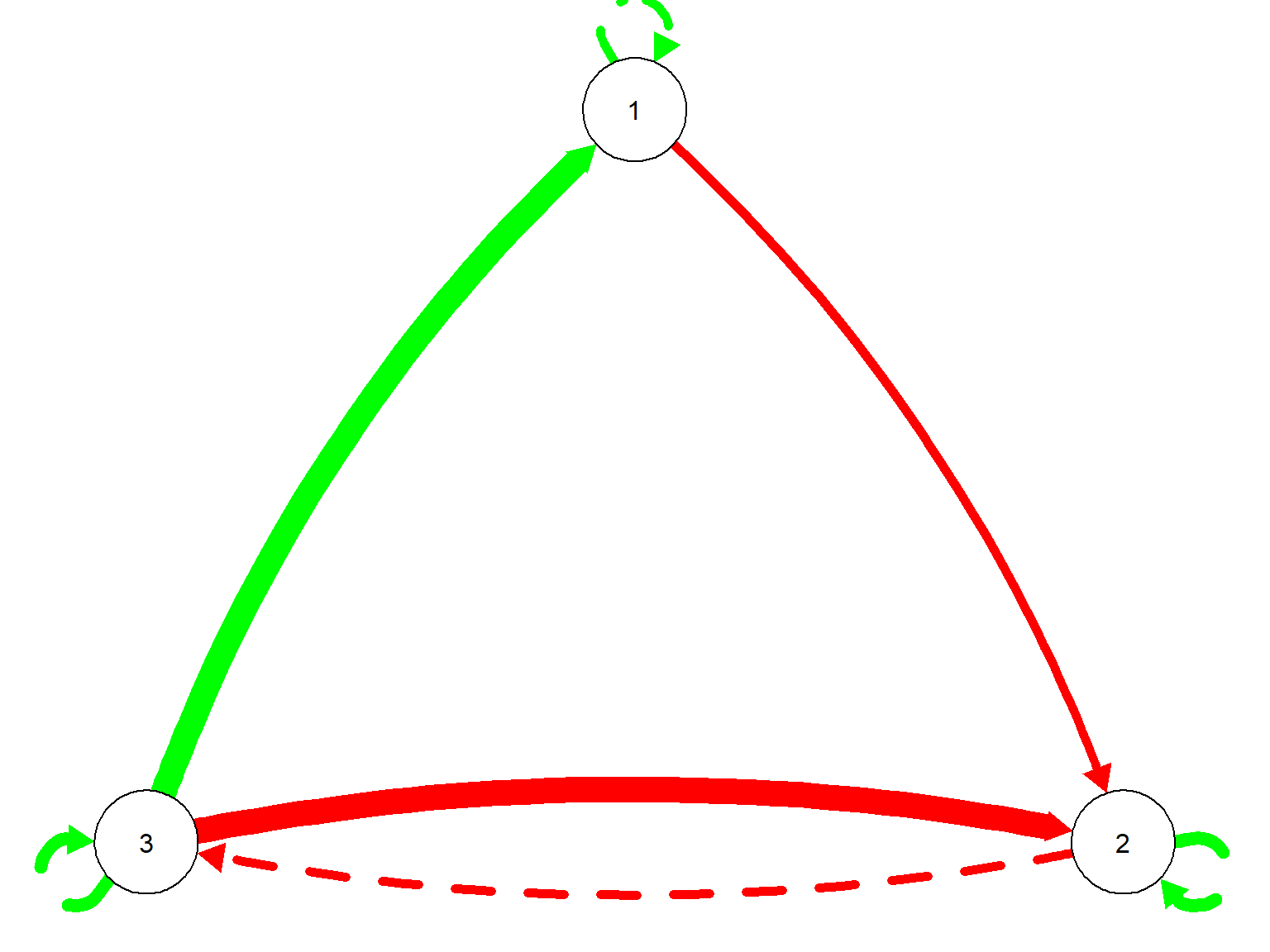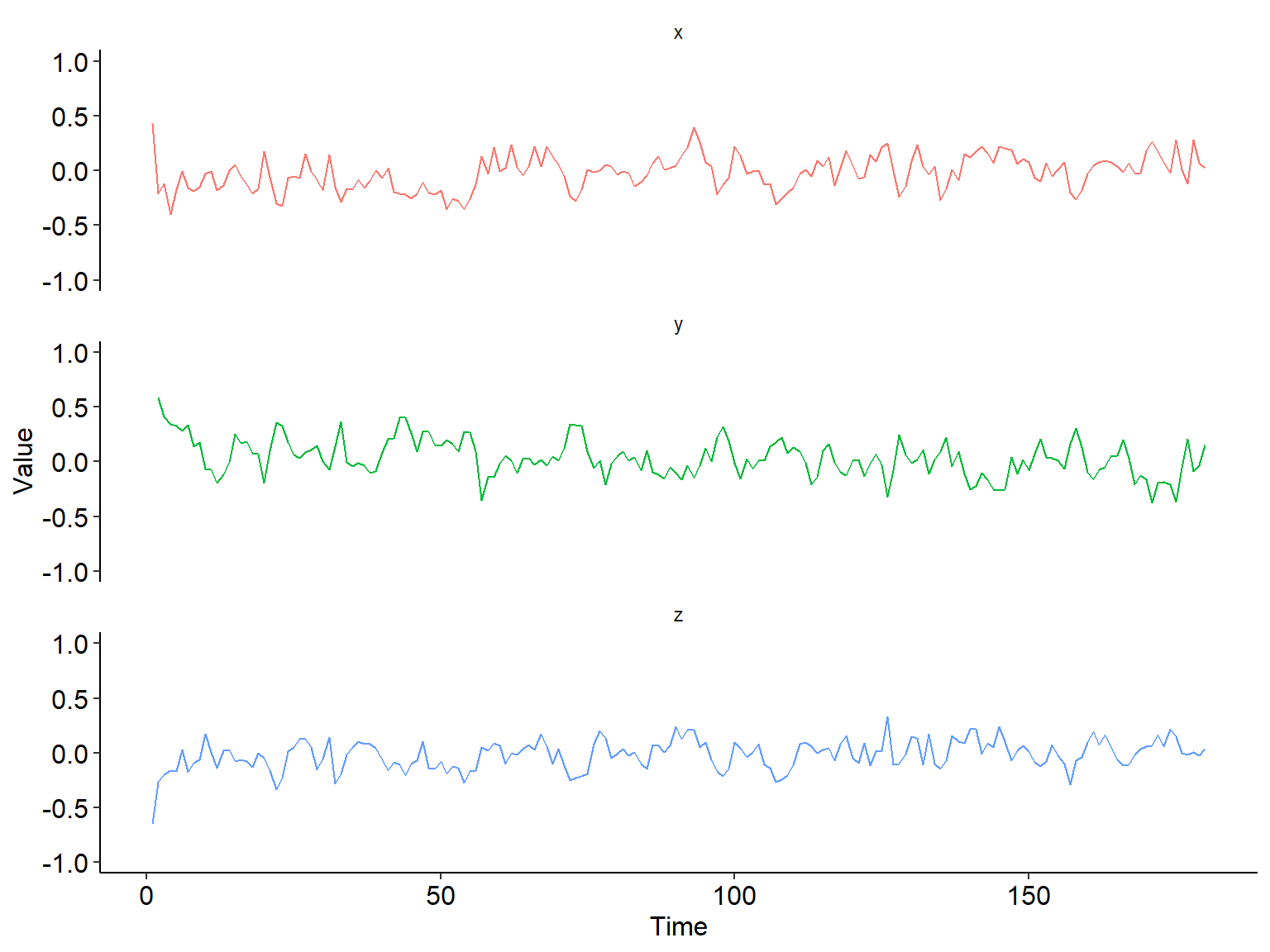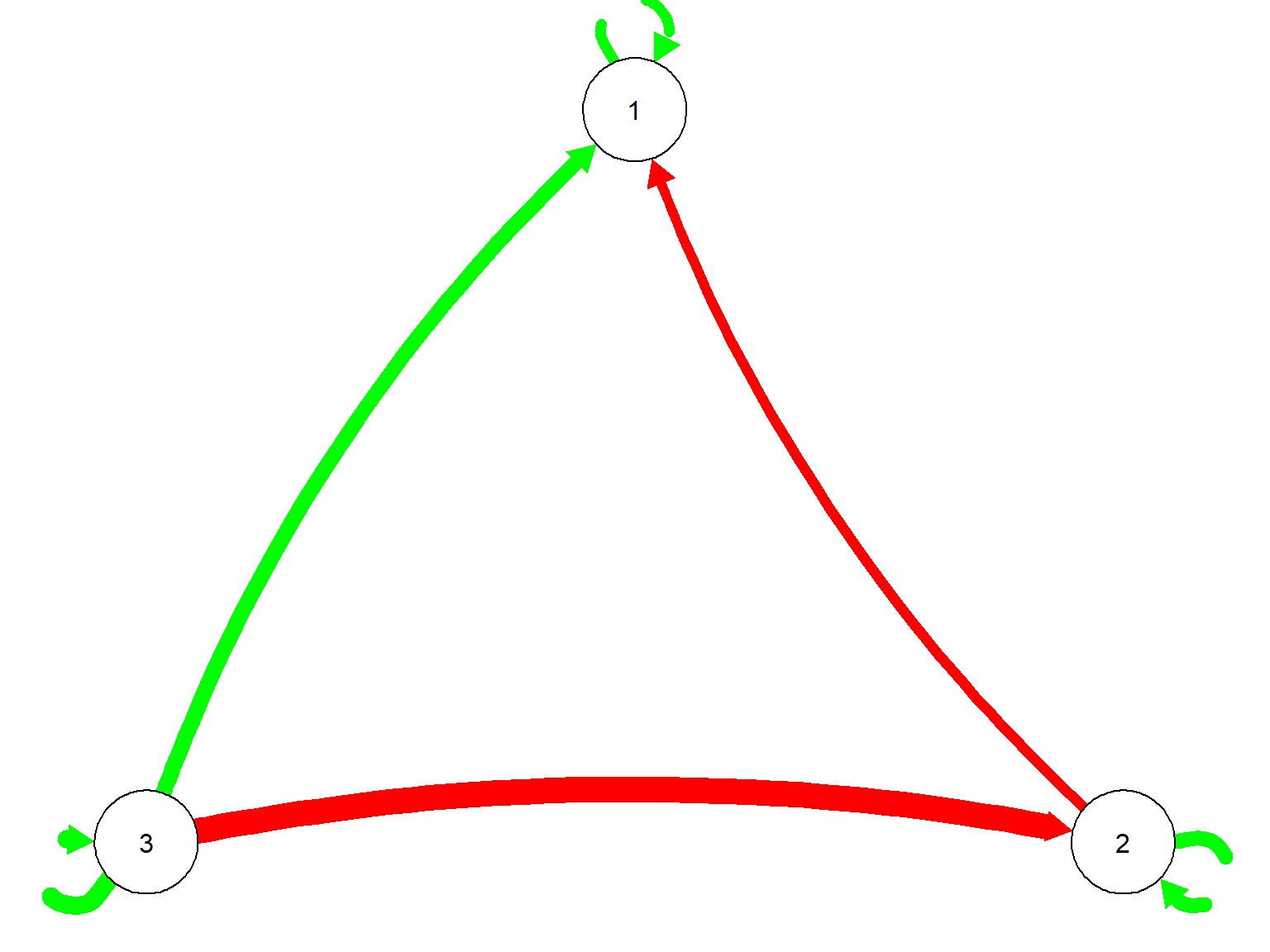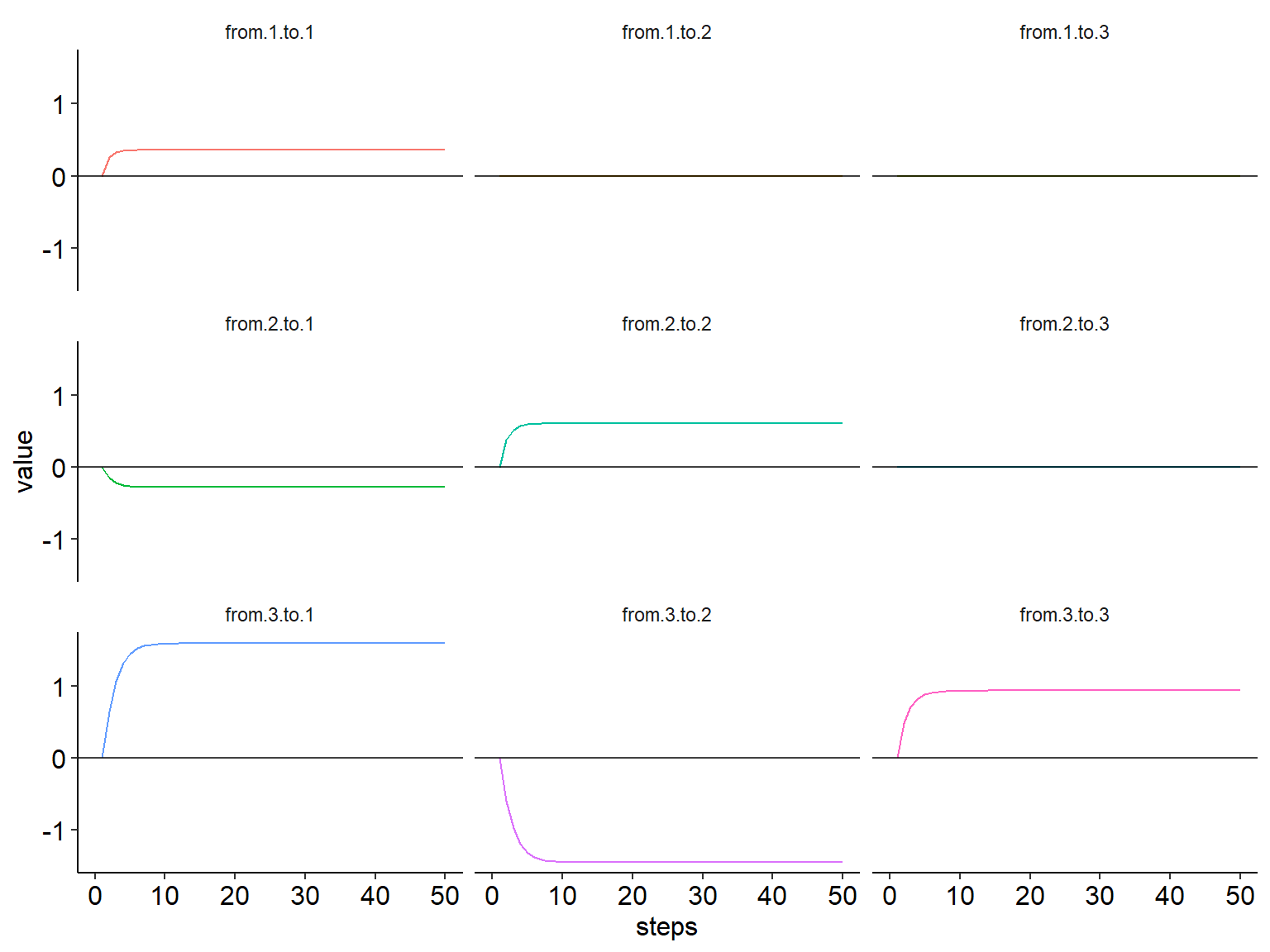### Overview

Network analysis has been increasingly adopted to analyze intensive longitudinal data. In this tutorial, we introduced a novel network-based metric, impulse response analysis matrix (iRAM), to extract information from the network. iRAM is built upon a sequential method that used dynamical system methods and impulse response analysis to model individuals differences in the system dynamics.

When using dynamical system methods to analyze intensive longitudinal data (e.g., ecological momentary assessment data, physiological data), we often conceptulize the multivariate system as an interconnected organism, where each variable at time t might affect itself or other variables at t+1. The dynamical system methods (e.g., vector autoregression models) are applied to estimate these temporal relations between variables. The interconnectedness leads to the depiction of these dynamical systems as networks.

One way to understand the network is to examine how far an external perturbation can derail the system. This is achieved by a technique called impulse response analysis (Lutkepohl, 2007). Impulse response analysis is especially useful when studying emotion-regulation, e.g., when a threat occurs, a person’s physiological system and subjective experience might respond in a concordant manner, so to produce a substantial increase of physiological arousal or recognition of stress to prepare the person to fight or flight from the threat. So the impulse in impulse response analysis mimicks the external perturbation on one variable, e.g., threat increased physiological arousal, and then through the intertwined network between the physiological system and subjective experience, this increase in arousal will filter through the network and reverberate in the network, causing the eventual observed result of sutained increase of physiological arousal or recognition of stress.

Data that can use this method are intensive longitudinal data, e.g., ecological momentary assessment (EMA), physiological measurements, neural activity, behavior codes.

This tutorial is also the summplementary material for the following paper: Yang, X., Ram, N., Lougheed, J.P., Molenaar, P.C.M., & Hollenstein, T. (2019). Adolescents’ Emotion System Dynamics: Network-based Analysis of Physiological and Emotional Experience. Developmental Psychology, 55(9), 1982-1993. DOI: 10.1037/dev0000690

To facilitate the replication and application of this approach, we have built a R package called “POMPOM” (POMPOM stands for Person-Oriented Method and Perturbation On the Model).

POMPOM is available on CRAN: https://CRAN.R-project.org/package=pompom.

For instructions on how to use the functions, see manual at: https://cran.r-project.org/web/packages/pompom/pompom.pdf.

Source code of POMPOM is at repository: https://github.com/vwendy/pompom.

### Outline of Tutorial

1. Prepare simulation of time-series data
2. Apply dynamical system method (unified Structural SEM, uSEM) on multivariate time-series data
3. Apply a network analysis method (also called impulse reponse analysis) on person-specific network and compute impulse response analysis matrix (iRAM)
library(pompom) # a package that extract network metric iRAM
library(reshape2) # data formating package
library(ggplot2) # data visualization package
library(qgraph) # network graph package
set.seed(1234) # set the seed for simulation 

### Step 1. Prepare simulation of time-series data

To demonstrate the analytical steps, we simulated a straightforward trivariate example. The readers can plug in their own intensive longitudinal data, in the long-format with each participant’s multiple variables.

#### Set up a three-node network

Time series was simulated based on the temporal relations and process noise. Our hypothetical 3-node newtork was using simulated data based on a pre-defined temporal relationship matrix and process noise. The temporal relationship is shown in true.beta with arbitrarily chosen parameters, and process noise is at Mean = 0, SD = .1.

Because in the paper, we used the difference score of time-series data of physiological data, we use the notation $$\Delta \eta$$ here.

$$\Delta \eta(t) = (I-\ A \ )^{-1}\Phi\Delta \eta(t-1) + (I-\ A \ )^{-1}\zeta(t)$$

where $$\ A$$ is the block of contemporaneous relations (southeast block of the beta matrix),

$$\Phi$$ is the block of lag-1 relations (southwest block of the beta matrix),

$$\zeta$$ is the process noise.

The three-node network is a hypothetical example used for the purpose of illustration. The numbers in true.beta matrix were arbitrarily chosen. As explained in the paper, the variables in time-series data are nodes and the temporal relations are edges, in the network terminology. We simulated 180 occasions to represent 180 repeated measurements of the three variables in the real studies.

Note here in the paper, we used the difference score of time-series data of physiological data, because the raw time-series data is non-stationary. Thus, the temporal relations in the paper refer to the dynamics among the difference scores of the raw physiological time-series data.

n.obs <- 180 # number of observation
p <- 3 # number of variables
noise.level <- 0.1
zeta <- cbind(rnorm(n.obs,0, noise.level),
rnorm(n.obs,0, noise.level),
rnorm(n.obs,0, noise.level)) # 3 time-series of noise for 3 variables

true.beta <-  matrix(c(0,0,0,0,0,0,
0,0,0,0,0,0,
0,0,0,0,0,0,
0.2,0,0.25,0,0,0.6,
0,0.3,0,-0.2,0,-0.6,
0,-0.2,0.3,0,0,0),
nrow = 2 * p,
ncol = 2 * p,
byrow = TRUE)

contemporaneous.relations <- matrix(true.beta[(p+1):(2*p),(p+1):(2*p)], nrow = p, ncol = p, byrow = F)
lag.1.relations <- matrix(true.beta[(p+1):(2*p),1:p], nrow = p, ncol = p, byrow = F)

True model is:

true.beta
##      [,1] [,2] [,3] [,4] [,5] [,6]
## [1,]  0.0  0.0 0.00  0.0    0  0.0
## [2,]  0.0  0.0 0.00  0.0    0  0.0
## [3,]  0.0  0.0 0.00  0.0    0  0.0
## [4,]  0.2  0.0 0.25  0.0    0  0.6
## [5,]  0.0  0.3 0.00 -0.2    0 -0.6
## [6,]  0.0 -0.2 0.30  0.0    0  0.0

#### Network graph

This is to visualize the temporal dynamics in the network form. To reiterate the terminologies of networks, each of the 3 variables is depicted as a node (circle), and the temporal relations among the nodes are depicted as edges (arrows, and the arrow indicate directionality of the temporal relationship), with color indicating sign of relation (green = positive, red = negative), thickness indicating strength of relation, and line shape indicating temporality of the association (dashed = lag-1, solid = contemporaneous). Lag-1 relations refer to the temporal relations between variables from measurement t - 1 to the measurement t, and contemporaneous relations refer to the temporal relations between variables within the same measurement.

plot_network_graph(true.beta, p)## NULL

#### Simulate time-series

time.series <- matrix(rep(0, p * n.obs), nrow = n.obs, ncol = p)
time.series[1,] <- rnorm(p,0,1)

row <- p
for (row in 2:n.obs)
{
time.series[row,] <- solve(diag(1,p, p) - contemporaneous.relations) %*%
lag.1.relations %*% time.series[row-1,] +
solve(diag(1,p, p) - contemporaneous.relations) %*% zeta[row, ]
}
time.series <- data.frame(time.series)
names(time.series) <- c("x", "y", "z")

#### Plot the simulated time-series

time.series$time <- seq(1,length(time.series[,1]),1) time.series.long <- melt(time.series, id="time") ## convert to long format ggplot(data=time.series.long, aes(x=time, y=value, colour=variable)) + geom_line() + facet_wrap( ~ variable , ncol = 1) + scale_y_continuous(breaks = seq(0, 100, by = 50)) + theme( strip.background = element_blank(), panel.background = element_blank(), legend.title = element_blank(), legend.key = element_blank(), legend.position = "none", axis.text.y=element_text(color="black",size=12), axis.text.x=element_text(color="black",size=12), axis.title.y=element_text(color="black",size=12), axis.title.x=element_text(color="black",size=12), axis.line = element_line(color = 'black') )+ ylim(-1,1)+ xlab("Time") + ylab("Value") ## Scale for 'y' is already present. Adding another scale for 'y', which ## will replace the existing scale. ## Warning: Removed 1 rows containing missing values (geom_path).time.series$time <- NULL

### Step 2. Apply dynamical system method (unified Structural SEM, uSEM) on the trivariate time-series data

#### Use uSEM to fit the model and parse parameters.

The model fit summary will give and estimates, which are essential information to conduct network analysis later. The model fit summary also gives the fit statistics.

For instructions on how to use the functions (e.g., “uSEM”), see manual at: https://cran.r-project.org/web/packages/pompom/pompom.pdf

var.number <- p # number of variables
lag.order <- 1 # lag order of the model

model.fit <- uSEM(var.number,
time.series,
lag.order,
verbose = FALSE,
trim = TRUE)

Now the uSEM model result is in the object “model.fit”, including beta matrix, psi matrix, and fit statistics. Next, we can the model.fit object into beta matrix and plot the estimated network graph.

beta.matrix <- parse_beta(var.number = p,
model.fit = model.fit,
lag.order = 1,
matrix = TRUE) # parse temporal relations in matrix format

plot_network_graph(beta.matrix$est, var.number)## NULL We can see here the estimated network recovered most of the network base on true beta (depicted in the first network graph), where a false negative was found (magnitude of -0.2, from node 2 to 3, lag-1 relation), and a direction of contemporaneous was flipped - from node 1 to node 2 was estimated as from node 2 to node 1 (magnitude of -0.2). #### Model fit Model fit should obey a 3 out of 4 rule (CFI and TLI should be at least .95, and RMSEA and SRMR should be no greater than .08). The “model_summary” function will return an object that contains beta, psi and fit statistics, shown in the following code chunks. mdl <- model_summary(model.fit, var.number, lag.order) mdl$cfi
##       cfi
## 0.9863237
mdl$tli ## tli ## 0.9813505 mdl$rmsea
##      rmsea
## 0.06524302
mdl$srmr ## srmr ## 0.08246155 We can see the estimated model has satisfying model fit. ### Step 3. Apply a network analysis method (also called impulse reponse analysis) on person-specific network and calculate impulse response analysis matrix (iRAM) Conceptually, impulse response analysis is to perturb the system (the whole emotion network we estimated) one node at a time. After the system receives such perturbation, or impulse, the impulse will reverberate through the network based on the network configuration. As aforementioned, in the manucript (Yang et al., 2019), we used the difference score of time-series data of physiological data, because the raw time-series data is non-stationary. Here, we compute the equilibrium of each time profile and plot the integrated form of time profiles, which is the trajectory of impulse response. iRAM_equilibrium_value <- iRAM_equilibrium(beta = mdl$beta,
var.number = var.number,
lag.order = lag.order)

iRAM_equilibrium_value

The variable name in “iRAM_equilibrium_value” consists of two numeric numbers, where the first one indicates to which node the perturbation is given, and the second one indicates for which node the equilibrium is computed. E.g., “e12” indicates the equilibrium of node 2 when node 1 is given perturbation.

#### Plot the integrated time profile

plot_integrated_time_profile(beta.matrix = mdl\$beta,
var.number = 3,
lag.order = 1)## NULL

In this plot, each row shows the responses of 3 nodes when only 1 node is given perturbation. E.g., “from.1.to.2” is the plot of time profile of node 2 when node 1 is given perturbation.

We can see that the equilibra in the integrated time profiles are consistent with the computed iRAM.